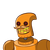# 1.If one side of a regular pentagon is 10 cm, then its perimeter is(a) 100 cm (b) 40 cm (c) 50 cm2. 5/2= September 8, 2021 by Serenity

1.If one side of a regular pentagon is 10 cm, then its perimeter is

(a) 100 cm (b) 40 cm (c) 50 cm

2. 5/2=

(a) 0.5
(b) 0.25
(c) 2.5

3.The rule which gives the number of matchsticks required to make matchstick pattern V, is

(a) 2n
(b) 3n
(c) 4n

5. The cost of 1 dozen pens is Rs. 24. Find the cost of 1 pen

(a) Rs.24
(b) Rs. 12
(c) Rs.2

5.Which of the following is an equation in a variable?

(a) x + 12 >19 (b) (5×2)- 7 = 3 (c) x-1 = 0​

### 1 thought on “1.If one side of a regular pentagon is 10 cm, then its perimeter is<br /><br />(a) 100 cm (b) 40 cm (c) 50 cm<br /><br />2. 5/2=<b”

1.